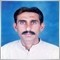# Experiments ... - page 57198037

As a natural continuation - ema (r-squared) adaptive (experiment) that has an option to be double smoothed too (in this case double smoothing is showing why is it so good so far - the values are hardly different, but they are much smoother than the original values)

Files:1202

As a natural continuation - ema (r-squared) adaptive (experiment) that has an option to be double smoothed too (in this case double smoothing is showing why is it so good so far - the values are hardly different, but they are much smoother than the original values)

Thanks for all the new mutants to play with, Dr. mladenstein :)[Deleted]
krelian99:
Thanks for all the new mutants to play with, Dr. mladenstein :)
:-) Dr. mladenstein :-) i must laugh...3231

krelian99:
Thanks for all the new mutants to play with, Dr. mladenstein :)
I second that :)45

As a natural continuation - ema (r-squared) adaptive (experiment) that has an option to be double smoothed too (in this case double smoothing is showing why is it so good so far - the values are hardly different, but they are much smoother than the original values)198037

A sort of an experiment too :

This is rsi (6 types of rsi) with automatic Fibonacci channels attached to it. Also price smoothing prior to rsi calculation is included (which makes it a rsioma too). Usual set of 22 prices types included.

PS: I think that from the example it is obvious that this indicator is metatrader 5 version (we can not have that type of display using metatrader 4)

Files:198037

And this is as experimental as it gets : this is T3 r-square adaptive made to be an oscillator. All the whistles and bells are at the right places, and maybe this representation helps a bit as far as the strength of momentum is concerned. We shall have to test in the following days if this way of converting an average (of any kind) can be used in real time trading successfully (and does it help us at all).

So : treat is as a total experiment for now till we have time to test it3231

And this is as experimental as it gets : this is T3 r-square adaptive made to be an oscillator. All the whistles and bells are at the right places, and maybe this representation helps a bit as far as the strength of momentum is concerned. We shall have to test in the following days if this way of converting an average (of any kind) can be used in real time trading successfully (and does it help us at all).

So : treat is as a total experiment for now till we have time to test it

Nice. Thanks :)4935

And this is as experimental as it gets : this is T3 r-square adaptive made to be an oscillator. All the whistles and bells are at the right places, and maybe this representation helps a bit as far as the strength of momentum is concerned. We shall have to test in the following days if this way of converting an average (of any kind) can be used in real time trading successfully (and does it help us at all).

So : treat is as a total experiment for now till we have time to test it

Thanks for well introduced (r-square adaptive) extension.

since you explained in a reply for applying this in new averages that it is not possible to applicable on all averages,do you think this will be applied individually on every ,where it is possible but this way there will be a long list of averages r-square versions ..... is there any chance for TEMA r-square ver ?

it was so best if found a universal r-square formula for newest averages - at least for 20/25 of totals.

regards198037

mntiwana:

Thanks for well introduced (r-square adaptive) extension.

since you explained in a reply for applying this in new averages that it is not possible to applicable on all averages,do you think this will be applied individually on every ,where it is possible but this way there will be a long list of averages r-square versions ..... is there any chance for TEMA r-square ver ?

it was so best if found a universal r-square formula for newest averages - at least for 20/25 of totals.

regards

mntiwana

Here is just part of problems that are happening with r-square adapting ...

This example is suing r-square adapting mode that is used in ema and t3 (the r-squared version) applied to linear regression value (or lsma as some call it) - period 50 - the same as the t3 example:

As you can see, it is a bit too "nervous" - which is strange at the least, since linear regression is in the exact same line of calculation as r-squared - at least 90% of the calculation is exactly the same, and then, in order to make it more usable,  I have to apply the "more conservative" way of r-square adapting (like this)

But that is not a solution either - short periods used for both calculations are a disaster and without applied some additional smoothing results are far from being usable.

Also, we can not have a code that must be adjusted manually every time when the results are not what we expect. For now, some averages are really resisting being r-square adaptive the way it is just fine for ema and t3. If I find some general solution, I will make it and post. In the mean time any other average will be posted as a part of testing and not as a part of a "solution" (I can not make a "new averages" and tell : "but now only 20 of 37 are adaptive"), so it all will probably be posted here as a part of ongoing experiments. Which means : no promises. We shall have to see what can we get